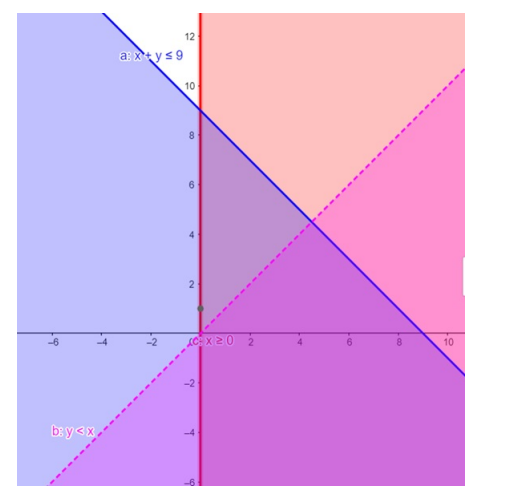# Solve the given inequalities

Question:

Solve the given inequalities $x+y \leq 9, y Solution: The graphical representation of$x+y \leq 9, y

$x+y \leq 9 \ldots \ldots$ (1)

$y$x \geq 0 \ldots \ldots$(3) Inequality (1) represents the region below line$x+y=9$(including the line$x+y=9$). Inequality (2) represents the region below line$x=y$(excluding the line$x=y$). Inequality (3) represents the region in front of line$x=0$(including the line$x=0\$ ).

Therefore,every point in the common shaded region including the points on the respective lines represents the solution for the given inequalities.

This can be represented as follows,